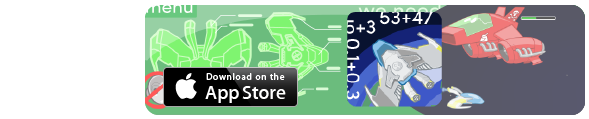# Twilight Take-Away

Calculate subtraction as difference by using The Bar Model.
LO:
Y2:To solve problems with (addition and) subtraction using concrete objects and pictorial representations, including those involving numbers, quantities and measures.
Y2:To recall and use addition and subtraction facts to 20 fluently, and derive and use related facts up to 100
Y2:To recognise and use the inverse relationship between addition and subtraction and use this to check calculations and solve missing number problems.
Y3:To add and subtract numbers mentally, including: a three-digit number and 1s, 10s (and 100s)
Y4:To solve number and practical problems that involve using knowledge of number & place value with increasingly large positive numbers

The fireflies have been collecting fairy dust. Work out the difference between how much the yellow flies collected and how much the green flies collected.

This game uses The Bar Model as an informal calculation strategy to illustrate subtraction as difference.

Have fun!

Made using theandlibraries.